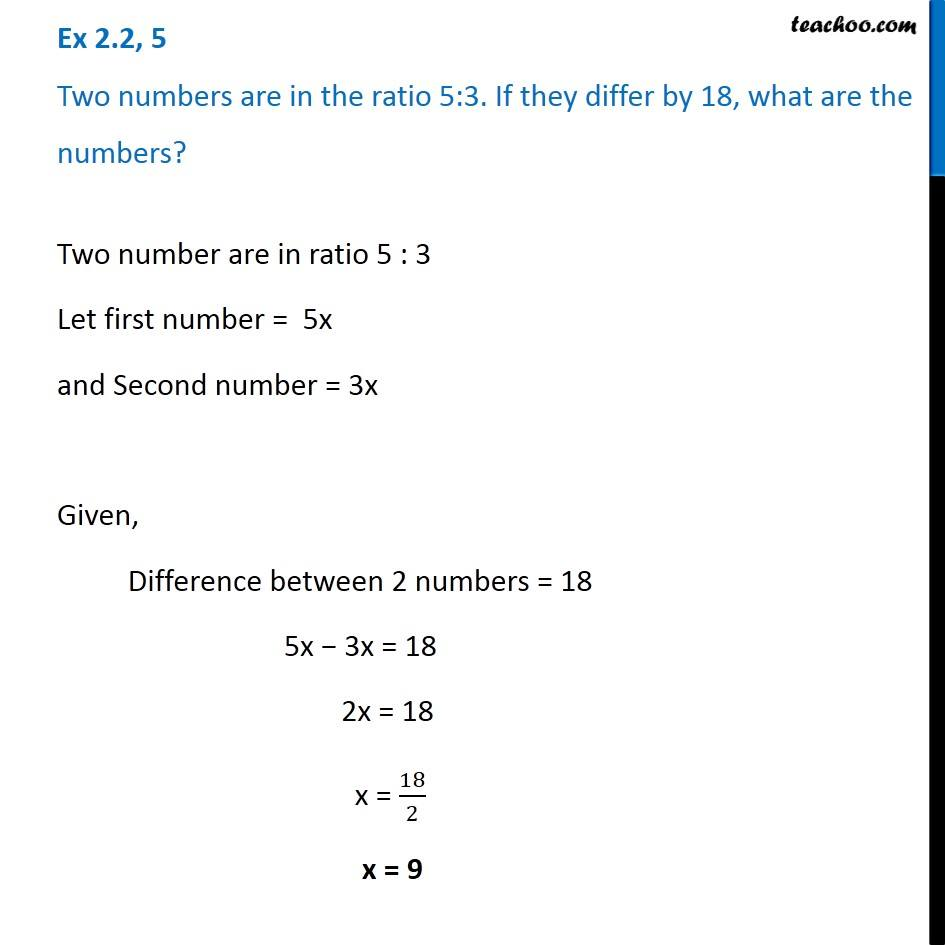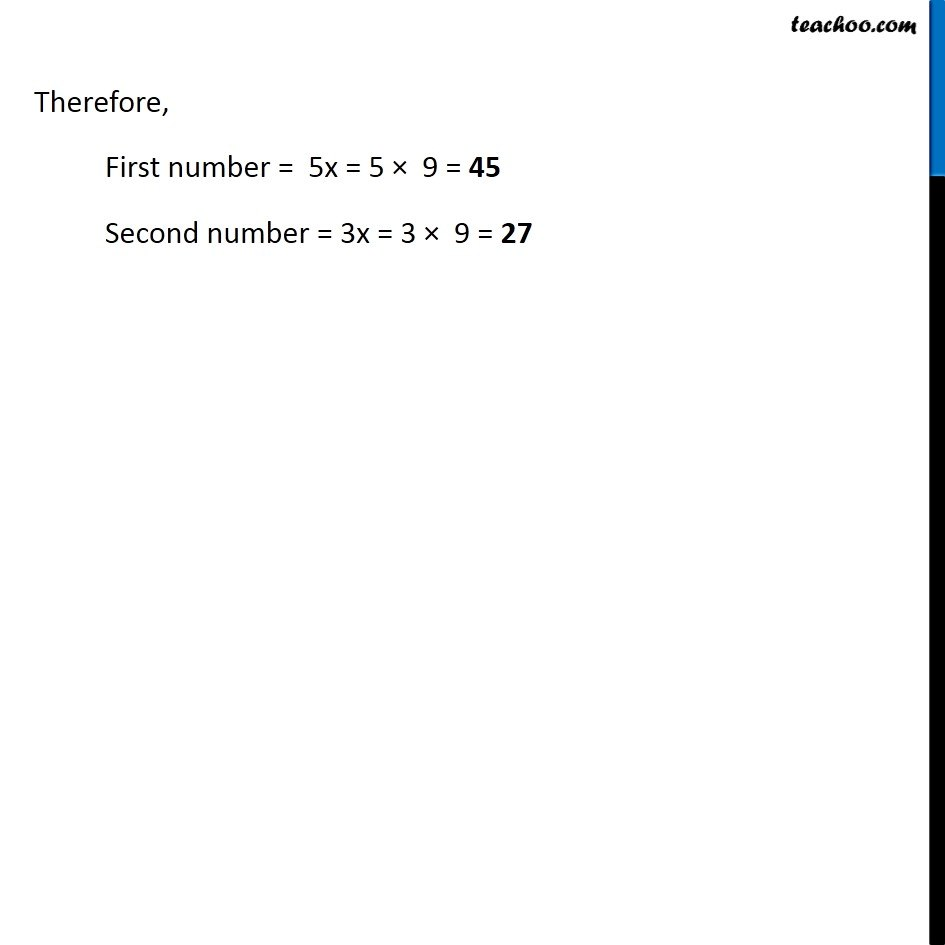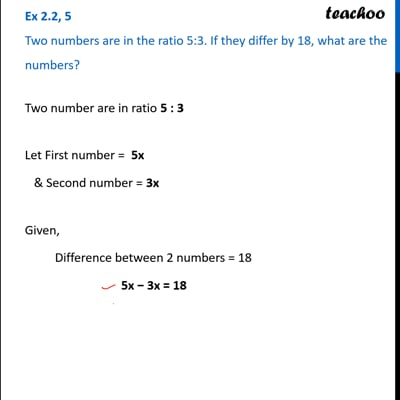Solving easy equations - Word Problems

Chapter 2 Class 8 Linear Equations in One Variable
Serial order wiseThis video is only available for Teachoo black users

Learn in your speed, with individual attention - Teachoo Maths 1-on-1 Class

### Transcript

Question 5 Two numbers are in the ratio 5:3. If they differ by 18, what are the numbers? Two number are in ratio 5 : 3 Let first number = 5x and Second number = 3x Given, Difference between 2 numbers = 18 5x − 3x = 18 2x = 18 x = 18/2 x = 9 Therefore, First number = 5x = 5 × 9 = 45 Second number = 3x = 3 × 9 = 27